Deep Learning is a new area of research on Machine Learning, which was introduced with the goal of bringing machine learning closer to one of its original goals: artificial intelligence.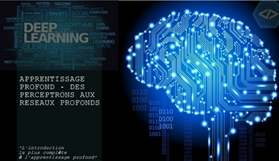Deep Learning is a new area of research on Machine Learning, which was introduced with the goal of bringing machine learning closer to one of its original goals: artificial intelligence.

Machine Learning?

It is a field of study of artificial intelligence, which is based on algorithms allowing giving to a machine the possibility of evolving in order to fill difficult or problematic tasks.

The goal is to create a machine capable of imitating a human spirit, and to do this, of course, it needs learning abilities.

However, it is not only about learning, it is about the representation of knowledge, reasoning and even thinking.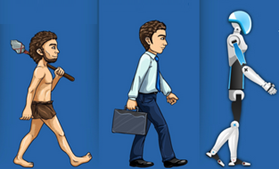Algorithms are used to analyze the data, and then make a determination or prediction about new data.

It is like using a regular program, writing an algorithm, the machine will run it on particular data, and then it could do the same task later with new data that it has never seen before.

In the definition, we focused on the fact of learning from data that are part of the Learning machine.

Rather than manually writing the code with a particular instruction set or task. The Learning machine is formed by using large amounts of data, so the algorithm gives it the ability to perform the task without showing how to do it.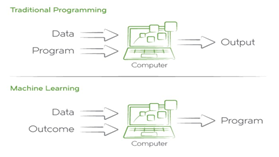How to do a spot using ML VS Traditional Programming?

Task: Sentiment Analyzer (analyze the feeling of a Media and classify it as positive or negative).

Traditional Programming: we give a list of words that could be classified as negative or positive, and we ask the algorithm to count how many times the words in the negative category compared to the words of the positive category in a particular article. Whoever is the longest will rate this article as positive or negative, of which we have the longest list.

Machine Learning: The algorithm would analyze large amounts of data from the given media and then deduce the characteristics

From these data and it would classify what a negative article looks like or positive.

The algorithm would learn words that are positive and negative. And later, we can give a new article that has never been seen before, and it could make a prediction that the articles are positive or negative, based on what he learned about the previous data.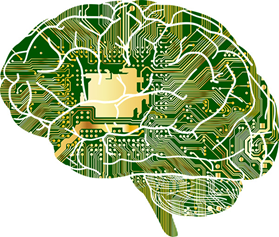Deep Learning?

DL is a ML subdomain that uses algorithms inspired by the structure and function of neural networks in the brain.

The structure of a neural network is like any other type of network; there is a network of interconnected nodes, called neurons, and edges that connect them together.

The main function of a neural network is to receive an input set, perform progressively complex calculations, and then use the outputs to solve a problem.

We always talk about algorithms that learn from data. It should be noted that learning will occur in supervised or unsupervised data.

Supervised learning occurs when the DL model learns and makes inferences from data that has already been tagged.

Unsupervised learning occurs when the model learns and makes inferences from unlabeled data.How can we identify if the image belongs to a cat or a dog?

When using supervised learning, we have a database of images of cats and dogs with different positions and each image will be labeled as a dog or cat, after we give these images to our model to have the possibility of knowing the image that belongs to a cat or a dog. It also allows to know the similarities and the differences between the two categories.

On the other hand, when unsupervised learning is used, the images are not labeled. The model will learn the features of the different images, after which it will categorize these images based on the similarities and differences without seeing their labeling.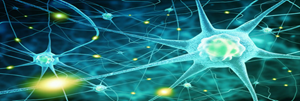Artificielle Neural Networks

ANNs are DL models that are based on the structure of brain neural networks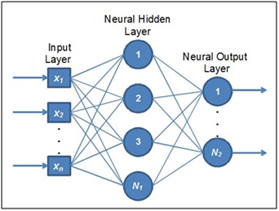A neural network is generally composed of a succession of layers each of which takes its inputs on the outputs of the previous except the neurons of the input layer. Each layer is composed of neurons, taking their inputs on the neurons of the previous layer.

Neurons are organized in layers:

• Input Layer.
• Hidden Layer.
• Output Layer.

In the example we find our first layer, which consists of the units, these units form our input layer, each of the units represents an individual characteristic of each sample in the set of data that will cross the model.

We can see that each of these inputs are connected to each unit of the next layer. The next layer is Hidden Layer simply because it is a layer between the input and output layers.

Each of these connections transfers output from the previous unit as input for the receiving unit. A connection from one unit to another will have its own WEIGTH assigned between 0 and 1.

WEIGHT represents the connection strength between the units, so the first input of the first layer, this when one receives input will go to the next unit via a connection, and will multiply with the WEIGTH assigned to that connection.

Weigthed SUM is calculated with each of the connections that point to these neurons. The sum is then passed to an activation function that converts the result into a number between 0 and 1. The result will be transmitted to the next neuron in the next layer.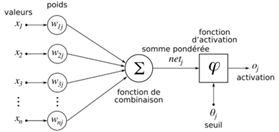Output = Activation (Weighted SUM of inputs)

During this process, the weights will continually change to achieve optimized weights on each connection, so the pattern will continue learning.

When we reach the last layer, Output Layer, the units represent the number of categories (in the example of the cat / dog we have two categories).

Activation or Transfer Function

The activation function of a neuron defines the output of this neuron according to a set of inputs.

It serves to introduce a non-linearity in the functioning of the neuron.

The thresholding functions generally have three intervals:

1. Below the threshold, the neuron is non-active (often in this case, its output is 0 or -1).
2. around the threshold, a transition phase.
3. Above the threshold, the neuron is active (often in this case, its output is 1).

Typical examples of activation functions are:

sigmoïde :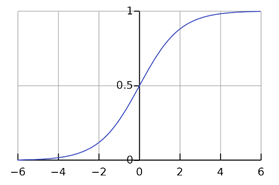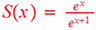f the input is a negative number, Sigmoid transferred this input to a number near the digit 0.

If the input is a positive number, it will be close to the number 1.

If the input is a number close to zero, the input will be between 0 and 1.

Function RELU: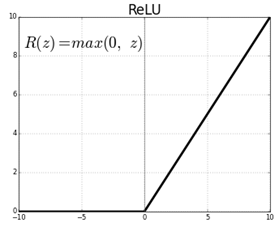Transform the input to a max of (0 or the same input).

If the input is less than 0 or equal to 0, RELU transforms the input to 0.

If the number is more than 0, RELU output what is given on Input.

Biologically inspired by the activity of our brain, where different neurons are triggered, or activated, by different stimuli.

How to practice Deep Learning?

In this part, we will talk about the prerequisites:

Python: We will need to be able to compile and execute Python code that uses TensorFlow for Deep Learning. Therefore, you have to install Python, Tensorflow and IDE (Jupyter).

1- Python:

It is possible to install and run Python / TensorFlow entirely from your own computer. Google provides TensorFlow for Windows, Mac, and Linux. Previously, TensorFlow did not support Windows. However, as of December 2016, TensorFlow supports Windows for CPU and GPU operation.

The first step is to install Python 3.6. In August 2017, this is the latest version of Python 3. I recommend using the Anaconda version of Python because it already includes many of the data science related packages that will be needed for this class. Anaconda directly supports Windows, Mac, and Linux. Download Anaconda from the following URL: https://www.anaconda.com/download/

2- Install a backend engine:

Theano, Tensorflow, or CNTK.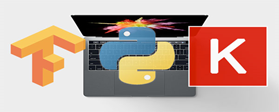3- Install Keras:

Keras is a high level Deep Learning API, written in Python. Keras uses TensorFlow by default, so if you are using Theano or CNTK, you will need to change the Keras configuration.

4- Install Python IDE: I propose you to work with Jupyter Notebook, a scientific notebook for python.

Commands to be executed at the prompt console «anaconda prompt":

``````conda install jupyter
jupyter notebook
conda install scipy
pip install sklearn
pip install pandas
pip install matplotlib
pip install pillow
pip install requests
pip install h5py
pip install tensorflow==1.4.0
pip install keras==2.1.2
``````

At the end, you have to execute the command: jupyter notebook in order to launch the notebook to reach the page above: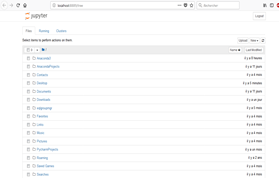To check the Keras / TensorFlow / Python version, you have to execute the code above to create a new notebook under Python.3:

``````import keras
import tensorflow as tf
import sys
import sklearn as sk
import pandas as pd
print("Tensor Flow Version: {}".format(tf.__version__))
print("Keras Version: {}".format(keras.__version__))
print()
print("Python {}".format(sys.version))
print('Pandas {}'.format(pd.__version__))
print('Scikit-Learn {}'.format(sk.__version__))
``````

In order to have the following result: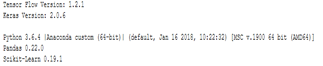author: LIMOUNI Anas.